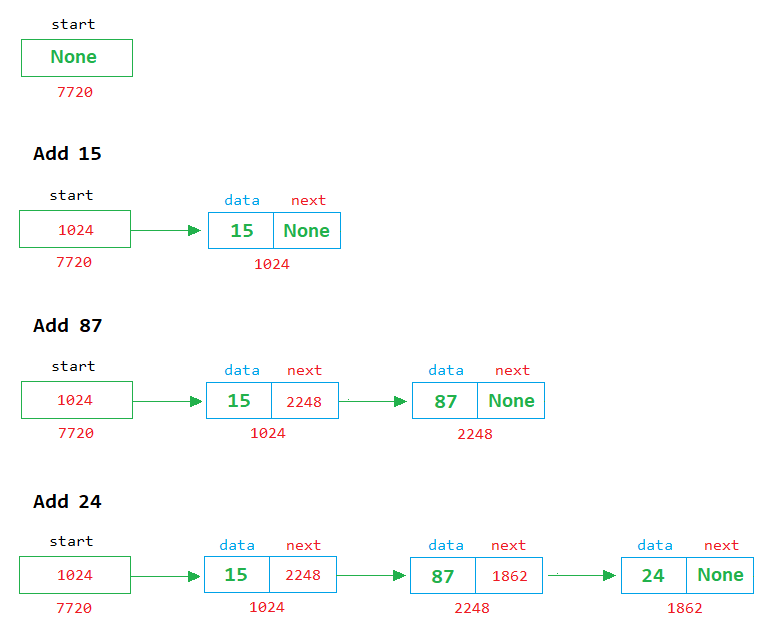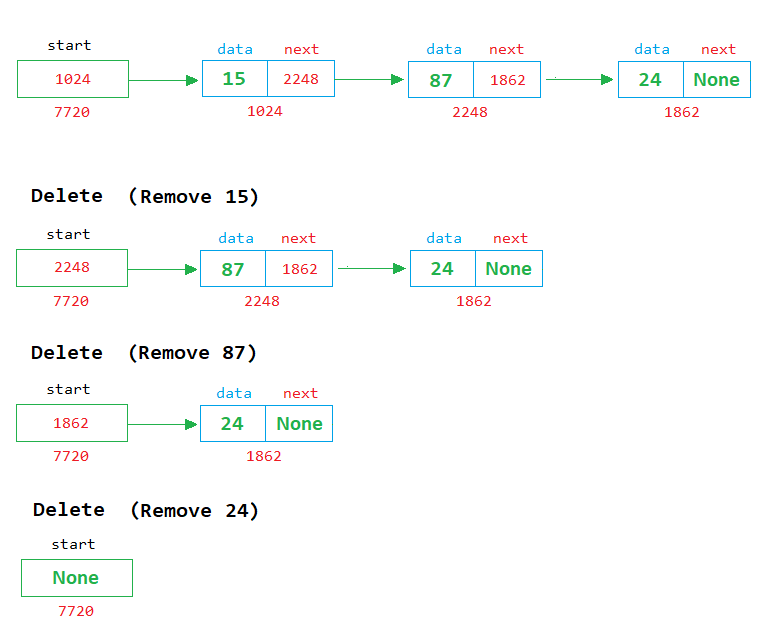# Queue using Linked List in Python

###### User-Defined Data Structures in Python

In this lesson, we will learn how to implement the queue using a singly linked list in python.

## Linked List Implementation of Queue in Python

We know about the queue and how to implement it using an array. This lesson will teach us how to implement the queue using a singly linked list.

We also know that two operations are possible on the queue, add and delete. See the image below to clearly understand how to implement add and delete operation on a queue using a linked list.In the above image, we can see that when a new node is created, it is linked with the last node.

### Delete ExampleIn the above image, we can see that each time a node is removed, the first node is deleted from the list, and the address of the next node is stored in the start variable.## Program of Queue using Linked List

Below is the complete program of queue in python using singly linked list having size 5.

#### Example

``````import os

# define node class
class Node:
def __init__(self):
self.data = 0
self.next = None

start = None
temp = None
rn = None
size = 5
top = 0
ch = None
n = None
c = 0

while 1:
os.system('cls')
print('2. Delete')
print('3. Display')
print('4. Exit')

if ch==1:   # for add operation

if c==size:
print('Queue is full')
input('Press enter to continue...')     # Pause the loop so that the user can see the message

else:
# Enter a number to store in node
n=int(input('Enter a number '))

# create a node object in temp
temp = Node()
temp.data = n           # assign the value of n in the data part of temp
temp.next = None        # assign the None in the next part of temp

if start == None:       # if start in None
start = temp        # then assign the temp in start (start points to the first node in the memory)

else:
rn = start          # if start is not None then assign start in rn to start reading from the first node

# run a while loop until we find None in the next part of rn
while rn.next is not None:
rn = rn.next    # if rn->next part is not None then move to the next node
rn.next = temp      # when while loop ends, the rn stands at the last node and we assign the temp in the next part of the rn
c=c+1

elif ch==2:     # for delete operation

if start==None:
print('Queue is empty')
input('Press enter to continue...')     # Pause the loop so that the user can see the message

else:
print('Number Deleted = %d' %(start.data))
temp=start
start=start.next
del(temp)
c=c-1
input('Press enter to continue...')     # Pause the loop so that the user can see the message

elif ch==3:     # for display operation

if start==None:
print('Queue is empty')
input('Press enter to continue...')     # Pause the loop so that the user can see the message

else:
temp=start		# start from 1st node
# display the nodes on the screen
while temp is not None:
print(temp.data, end=' ')
temp=temp.next
input('\nPress enter to continue...')     # Pause the loop so that the user can see the queue

elif ch==4:
exit(0)

else:
print('Wrong Choice')
input('Press enter to continue...')     # Pause the loop so that the user can see the message
``````